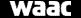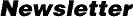Volume 12, Number 3, Sept 1990, pp.11-12

# A Solution to Solutions? Some Elementary Guidelines

by Tom Collings

(Reprinted in WAAC Newsletter from Paper Conservation News, No. 50 (June 1989), pp. 10-11, with permission of the author and The Institute of Paper Conservation, Leigh Lodge, Leigh, Worcestershire WR6 5LB, England.)

10% w/v, 15% w/w, 20% v/v, 15g/l, 4M. Just what do all these mean? It is surprising what a muddle some people get into with making up quite simple solutions, but really it is not that difficult.

The first thing to get established is what all these terms really mean. If we make a solution by dissolving 1 level teaspoon of a powder in half a pint of water we are measuring both in terms of volume. Thus the resultant strength of the solution is expressed with the term volume per volume, i.e. v/v. If, however, we take 2 ounces of powder and dissolve it in a gallon of water then one ingredient is measured by weight and the other by volume. So the strength of the solution is weight per volume, i.e. w/v. If we now make up a solution with 5 grammes of powder and 100 grammes of liquid both measures are by weight so that the solution is weight by weight, i.e. w/w.

When solutions are made up accurately by dissolving a solid in a liquid the solid is first dissolved in a small quantity of the liquid and the volume made up to the required level using a volumetric flask. With the solutions we use in conservation we don't usually need this degree of accuracy but what must not be done is to dissolve 10 grammes of solid in 100 cubic centimetres of liquid to give a 10% solution--less than 100 cubic centimetres of liquid will be needed to give 100 cubic centimetres of solution because of the expansion of the liquid when the solid is dissolved in it.

When it comes to percentages, working in teaspoonful, pints, or ounces will cause considerable difficulties. Such difficulties do not occur if all the measurements, whether by weight or volume, are measured using the metric system. The term "percentage" refers to parts per 100, the 100 referring to the final mixture, not one of the ingredients. This means that we need to dissolve 5 grammes of powder in 95 grammes of liquid to make 100 grammes of solution, which would then give us a 5% w/w solution. It is important that the units be consistent, either in grammes or cubic centimetres and the final mixture out of either 100 grammes or cubic centimetres. Thus, if 15 grammes of powder are dissolved in 1 litre of liquid, we have 15 parts by weight of powder to 1,000 parts by volume of liquid, so the solution is 1.5% w/v.

Problems can arise when liquids are sometimes measured by weight and sometimes by volume. Let's take an example: if we dissolve 1 gramme of powder in 99 grammes of water we have a 1% w/w solution. But 99 grammes of water has a volume of 99 cubic centimetres so we could, with the same quantities, express the concentration of the solution as 1% w/v. This works only with water. If we dissolve 1 gramme of powder in 99 cubic centimetres of ethanol to produce 100 cubic centimetres of solution we have again a 1% w/v solution. But 99 cubic centimetres of ethanol weigh 80 grammes not 99 grammes so the concentration of the solution expressed as w/w would be (1 x 100)/(1 + 80); i.e., 100/81=1.2% w/w.

A further complication often comes with the symbols used for the quantities, volume being sometimes very confusing. The old symbol for one cubic centimetre used to be cc, this was changed to ml (millilitre) and is now cm3. Furthermore there is a trend to use the unit dm3 (cubic decimetre) instead of litre. The symbol used for grammes has also changed. It used to be gm or gms (and sometimes gr or grs is found) but now it is simply g for both the singular and the plural. However, because many of the reference books we use are not modern and are often written by nonscientists, we must be aware of the possible variations.

If we wish to make up a 10% w/v solution of magnesium bicarbonate (magnesium hydrogen carbonate to give it its correct name) we cannot obtain it by dissolving magnesium bicarbonate powder in the appropriate volume of water because it does not exist outside an aqueous solution. So we need to do some calculations. Magnesium bicarbonate is 1produced in solution by bubbling carbon dioxide through a suspension of magnesium carbonate. The equation for the reaction is--

MgCO3 + CO2 + H2o=Mg(HCO3)2

We need to calculate the weight of magnesium carbonate required to produce 10 grammes of magnesium bicarbonate. To do this we must work out the molecular weights (relative molecular masses) of the two compounds, because this gives us the required figure for the calculation. To work out the molecular weights we need to know the atomic weights (relative atomic masses) of the elements involved. These are Magnesium 24, Hydrogen 1, Oxygen 16, Carbon 12. The molecule of magnesium carbonate has the formula MgC03. This means it consists of one Magnesium atom, one Carbon atom and three Oxygen atoms. This gives us a molecular weight of (1 x 24) + (1 x 12) + (3 x 16) i.e. a total of 84. The molecule of magnesium bicarbonate has a formula of Mg(HCO3)2. This means it consists of one Magnesium atom and two of (one Hydrogen atom + one Carbon atom + three Oxygen atoms), a total of one Magnesium atom, two Hydrogen atoms, two Carbon atoms and six Oxygen atoms, giving us a molecular weight of (1 x 24) + (2 x 1) + (2 x 12) + (6 x 16) i.e. a total of 146.

Because in the balanced reaction above one molecule of magnesium carbonate produces one molecule of magnesium bicarbonate, the weights of the compounds in the reaction are in direct proportion to their molecular weights. So 146 g of magnesium bicarbonate are produced in the reaction by 84 g of magnesium carbonate. Therefore it follows that if we want to produce 10 g of magnesium bicarbonate we need to use 10 x 84/146 g of magnesium carbonate i.e. 5.7 g. So if 5.7 g of magnesium carbonate are suspended in water, carbon dioxide passed through until it has all reacted and the final solution made up to 1 litre, we would have a concentration of magnesium bicarbonate of 10 g/l, that is, a 1% w/v solution.

Often the term molarity of solution is used, for example 4 molar (4M). A molar solution is one which contains the molecular weight of the substance in grammes per litre of solution. So it is necessary to work out molecular weights again. For example if we require a 4M solution of sodium hydroxide we first need to know that the formula is NaOH. It consists of one atom of Sodium (atomic weight 23) one atom of Oxygen and one atom of Hydrogen. (1 x 23) + (1 x 16) + (1 x 1) = 40. So a molar solution contains 40 g/l of sodium hydroxide and hence a 4M solution contains 160 g/l.

Sometimes it is required to make up a solution where the compound can exist in slightly different forms, one being anhydrous (without water) and others having water of crystallisation. A good example is barium hydroxide which can exist in the anhydrous form with a molecular formula of Ba(OH)2 or hydrated as Ba(OH)2.5H2O. To determine the factor to convert one to the other we again need to work out the molecular weights. Ba(OH)2 is 171 (ba=137) and Ba(OH)2.5H2O is 261 so the conversion factor is either 171/261, 0.66 for crystalline to anhydrous and 261/171, 1.53 for anhydrous to crystalline.

Concentrations of hydrogen peroxide solutions are sometimes quoted in molarity, more often in "volume strength." Volume strength is unique to hydrogen peroxide. When hydrogen peroxide decomposes it is converted to water with the liberation of oxygen gas. One litre of "10 volume" hydrogen peroxide will produce 10 litres of oxygen when it decomposes; and one litre of "5 volume" hydrogen peroxide will produce 5 litres of oxygen. So it is simply a convenient way of expressing the solution's strength. But remember that hydrogen peroxide solutions are not stable and decompose easily so that what has been bought as " 10 volume" does not stay at that strength for long.

Another class of often used compounds which are not stable are the hypochlorites--bleaching powder and sodium hypochlorite. (We won't mention Chloramine T because I hope you have stopped using it!) While it is common practice to use certain w/v percentage solutions, this is very unreliable. It is much better to use the percentage "available chlorine" expression of concentration and to check the actual strength of your solution before you use it. You can find details of this in the article "A simple method to determine the real strengths of hypochlorite solutions" (Paper Conservation News, No. 30, 1984).

Well, I think that covers most of it. I hope you have found it a help. I am constantly surprised at the problems some conservators have in making up solutions and perhaps now the solution will not be insoluble.

URL: http://
Timestamp:
Retrieved: